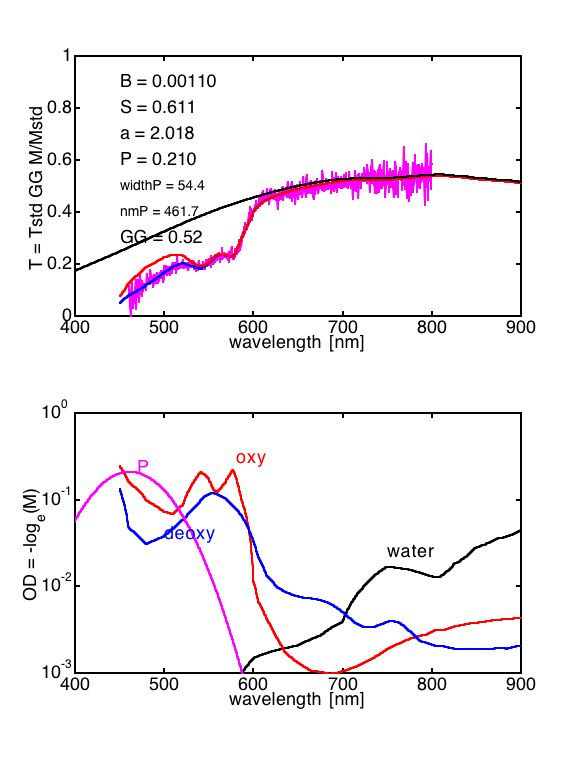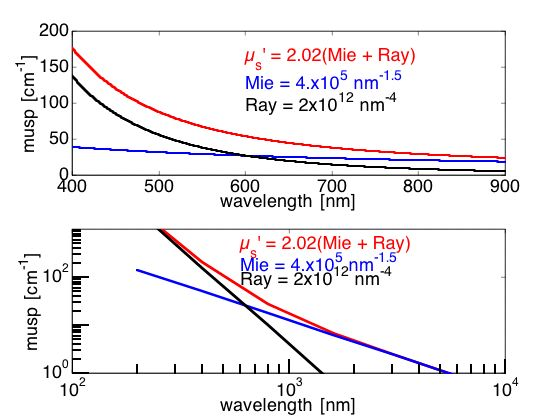# Summary of results

The result of fitting the spectral measurement of skin is summarized in the following figures that show the absorption coefficient (μa) and reduced scattering coefficient (μs') versus wavelength.Figure: (TOP) The final fit of the spectra. (BOTTOM) The absorption coefficients for oxy- and deoxy-blood, water, and bilirubin (porphyrin).Figure: The scattering coefficient spectrum. (TOP) Linear plot. (BOTTOM) Semi-logarthmic plot. The scattering coefficient is composed of both Mie-scattering and Rayleigh-scattering components.